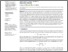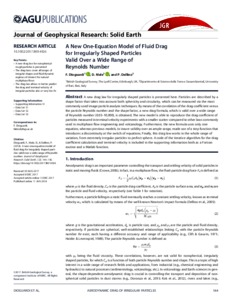nerc.ac.uk

# A New One-Equation Model of Fluid Drag for Irregularly Shaped Particles Valid Over a Wide Range of Reynolds Number

Dioguardi, F.; Mele, D.; Dellino, P.. 2018 A New One-Equation Model of Fluid Drag for Irregularly Shaped Particles Valid Over a Wide Range of Reynolds Number. Journal of Geophysical Research: Solid Earth, 123 (1). 144-156. https://doi.org/10.1002/2017JB014926Preview
Text
Dioguardi_et_al-2018-Journal_of_Geophysical_Research__Solid_Earth.pdf - Published Version

## Abstract/Summary

A new drag law for irregularly shaped particles is presented here. Particles are described by a shape factor that takes into account both sphericity and circularity, which can be measured via the most commonly used image particle analysis techniques. By means of the correlation of the drag coefficient versus the particle Reynolds number and the shape factor, a new drag formula, which is valid over a wide range of Reynolds number (0.03–10,000), is obtained. The new model is able to reproduce the drag coefficient of particles measured in terminal velocity experiments with a smaller scatter compared to other laws commonly used in multiphase flow engineering and volcanology. Furthermore, the new formula uses only one equation, whereas previous models, to insure validity over an ample range, made use of a step function that introduces a discontinuity at the switch of equations. Finally, this drag law works in the whole range of variation, from extremely irregular particles to perfect sphere. A code of the iterative algorithm for the drag coefficient calculation and terminal velocity is included in the supporting information both as a Fortran routine and a Matlab function.

Item Type: Publication - Article https://doi.org/10.1002/2017JB014926 21699313 10 Apr 2018 15:34 +0 (UTC) https://nora.nerc.ac.uk/id/eprint/519792View Item